# Problems of the Week

Contribute a problem

$5, 7, 17,$ and $19$ are four prime numbers such that the sum of any three of them is also prime: \begin{aligned} 5+7+17&=29\\ 5+7+19&=31\\ 5+17+19&=41\\ 7+17+19&=43. \end{aligned} Are there 5 distinct positive primes such that the sum of any three of them is also prime?

A space probe is on a circular orbit around the sun at a distance of $r_0 = 1 \,\text{AU}$ (astronomical unit). It collides with an asteroid in its trajectory, losing all of its kinetic energy. As a result, the space probe falls directly towards the sun.

How long (in days) does it take for the space probe to arrive at the sun? Round to the nearest integer.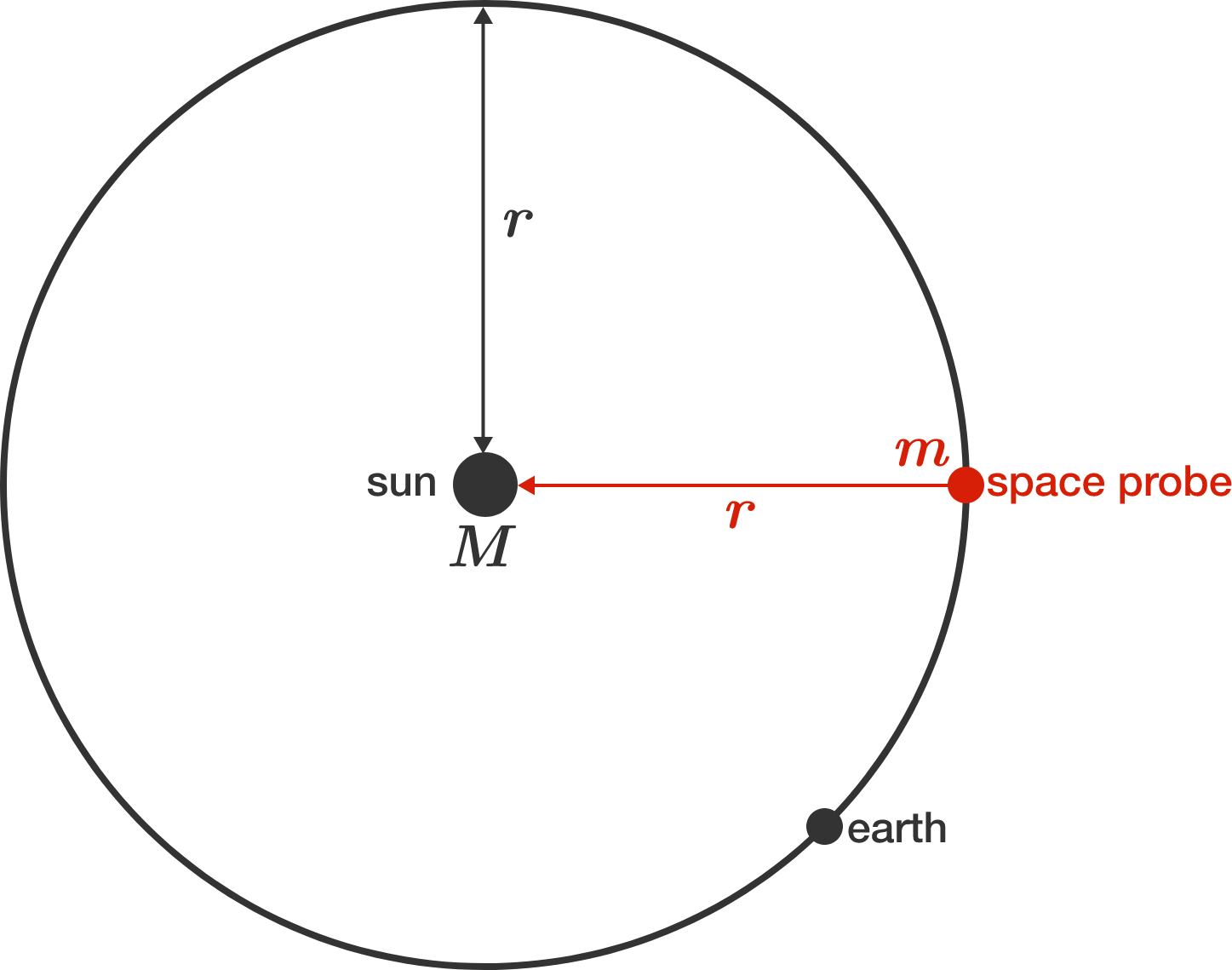Details and Assumptions: The Earth orbits the sun in an elliptical orbit with semi-major axis $1 \text{ AU},$ and has an orbital period of 365.25 days. The planets (including the Earth) have a negligible impact on the orbit of the probe. The diameter of the sun is negligible compared to the orbital radius $r_0.$

Two real numbers $x$ and $y$ are chosen uniformly at random on the interval $(0,1).$

What is the probability that the closest integer to $\frac xy$ is even?

Two spheres of radii $2$ and $8$ have their centers separated by a distance of $18.$

If $a\pi$ is the largest surface area that can be illuminated by a light source placed somewhere on the line joining their centers, what is $a?$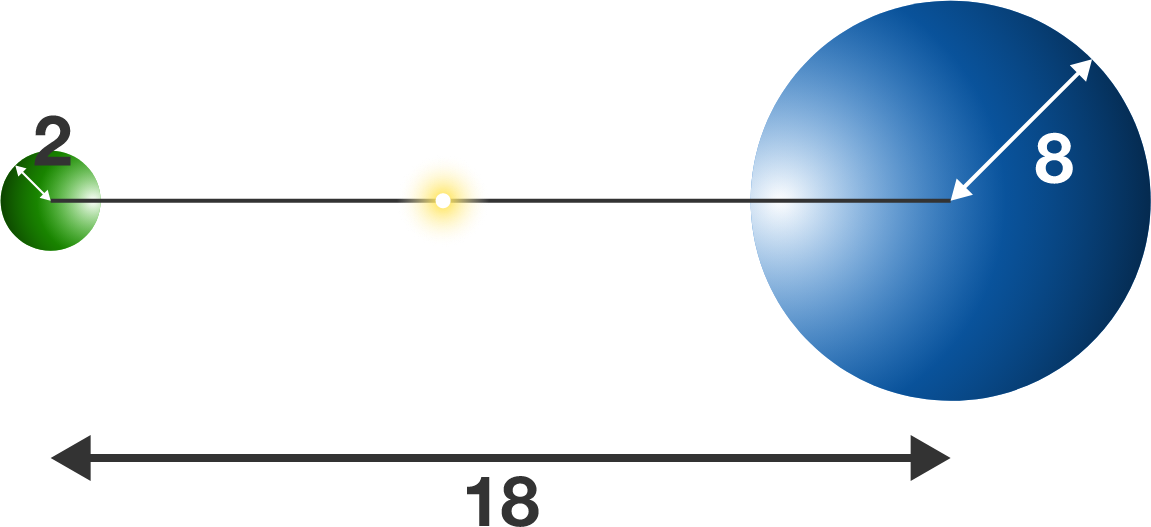The lower left edge of a cube has two opposite faces (the top and rightmost) with which it doesn't share a vertex. This is also true for the other 11 edges.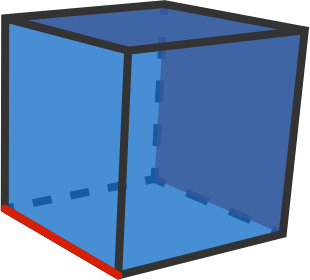We first cut off a wedge with a plane going through the bottom left edge and the line segment dividing the top face into two equal halves. We do the same for the other opposite face at right.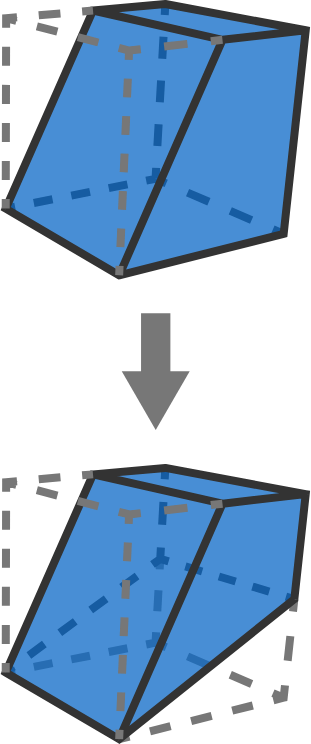Since the cube has 12 edges, we need a total of $2\times 12 =24$ straight cuts as described above to come up with the polyhedron shown below. If the volume of this core polyhedron is $V_P,$ and the volume of the original cube is $V,$ then $V_P = \frac{1}{n} V.$

What is $n?$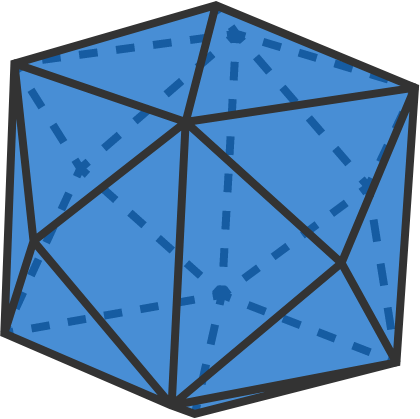×